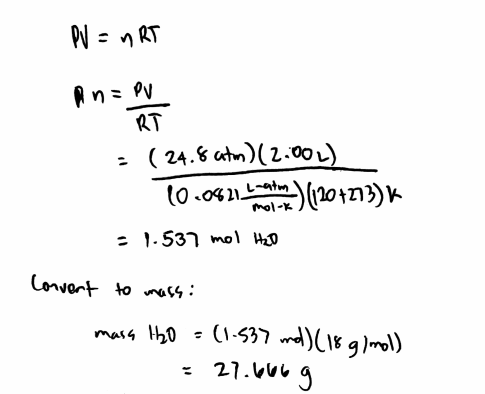# Problem: A certain hydrate has the formula MgSO4•xH2O. A quantity of 54.2 g of the compound is heated in an oven to drive off the water. If the steam generated exerts a pressure of 24.8 atm in a 2.00-L container at 120°C, calculate x.

###### FREE Expert Solution
84% (415 ratings)
###### FREE Expert Solution84% (415 ratings)###### Problem Details

A certain hydrate has the formula MgSO4•xH2O. A quantity of 54.2 g of the compound is heated in an oven to drive off the water. If the steam generated exerts a pressure of 24.8 atm in a 2.00-L container at 120°C, calculate x.

What scientific concept do you need to know in order to solve this problem?

Our tutors have indicated that to solve this problem you will need to apply the The Ideal Gas Law: Molar Mass concept. You can view video lessons to learn The Ideal Gas Law: Molar Mass. Or if you need more The Ideal Gas Law: Molar Mass practice, you can also practice The Ideal Gas Law: Molar Mass practice problems.

What is the difficulty of this problem?

Our tutors rated the difficulty ofA certain hydrate has the formula MgSO4•xH2O. A quantity of ...as high difficulty.

How long does this problem take to solve?

Our expert Chemistry tutor, Dasha took 9 minutes and 45 seconds to solve this problem. You can follow their steps in the video explanation above.

What professor is this problem relevant for?

Based on our data, we think this problem is relevant for Professor Bindell's class at UCF.